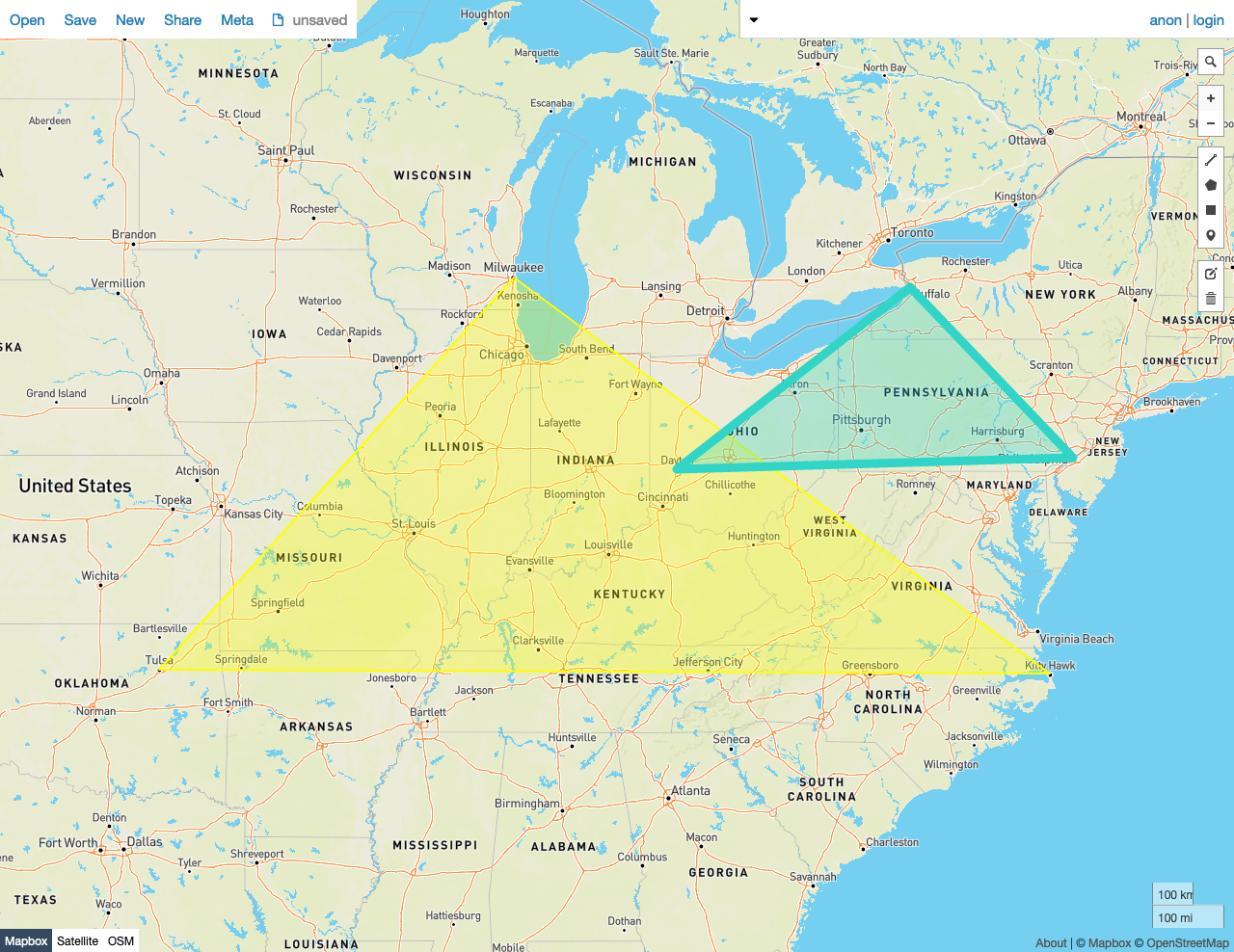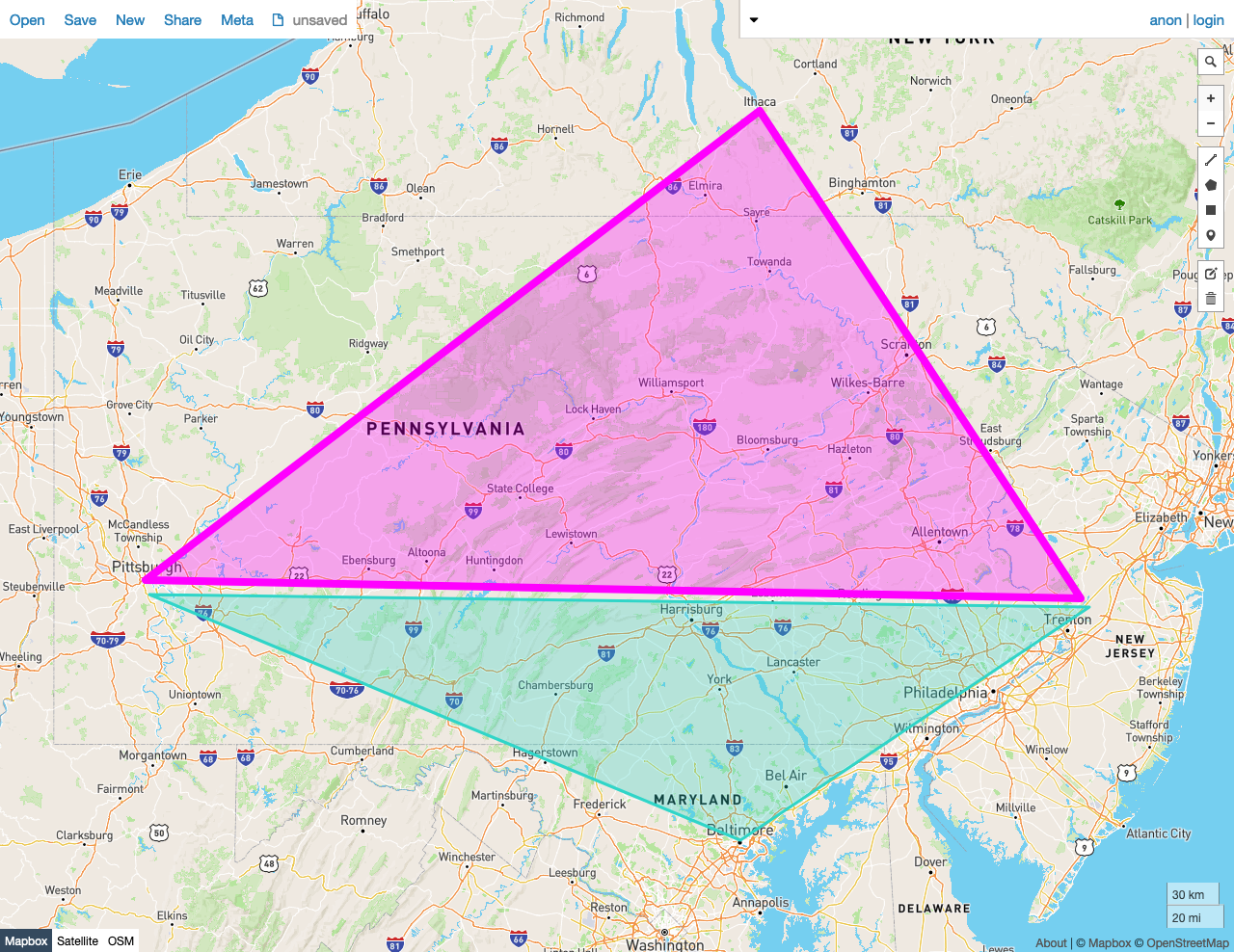On this pageWarning:
CockroachDB v20.2 is no longer supported. For more details, see the Release Support Policy.

Given two shapes A and B, `ST_Overlaps(A, B)` returns `true` if the following criteria are met:

• The shapes share any of the same space -- that is, if any point in the set that comprises A is also a member of the set of points that make up B.
• Neither of the shapes is contained by the other, in the `ST_Contains` sense.

In other words, `ST_Overlaps` returns `true` if the shapes intersect (in the `ST_Intersects` sense), but neither is contained within the other.

`ST_Overlaps` works on the following spatial data types:

Note:

`ST_Overlaps` will attempt to use any available spatial index to speed up its operation. Use the prefixed variant `_ST_Overlaps` if you do not want any spatial indexes to be used.

## Examples

Note:

The screenshots in these examples were generated using geojson.io, but they are designed to showcase the shapes, not the map. Representing `GEOMETRY` data in GeoJSON can lead to unexpected results if using geometries with SRIDs other than 4326 (as shown below).

### True

In this example, `ST_Overlaps` returns `true` because:

• There is a Point in the set that comprises Polygon A that is also a member of the set of Points that make up Polygon B.
• Neither of the shapes is contained by the other, in the `ST_Contains` sense.
``````SELECT st_overlaps(st_geomfromtext('SRID=4326;POLYGON((-87.906471 43.038902, -95.992775 36.153980, -75.704722 36.076944, -87.906471 43.038902))'), st_geomfromtext('SRID=4326;POLYGON((-84.191605 39.758949, -75.165222 39.952583, -78.878738 42.880230, -84.191605 39.758949))'));
``````
``````  st_overlaps
---------------
true

(1 row)
``````### False

In this example, `ST_Overlaps` returns `false` because:

• - There is not any Point in the set that comprises Polygon A that is also a member of the set of Points that make up Polygon B.
``````SELECT st_overlaps(st_geomfromtext('SRID=4326;POLYGON((-79.995888 40.440624,-74.666728 40.358244, -76.5 42.443333, -79.995888 40.440624))'), st_geomfromtext('SRID=4326;POLYGON((-79.976111 40.374444, -74.621157 40.323294, -76.609383 39.299236, -79.976111 40.374444))'));
``````
``````  st_overlaps
---------------
false
(1 row)
``````